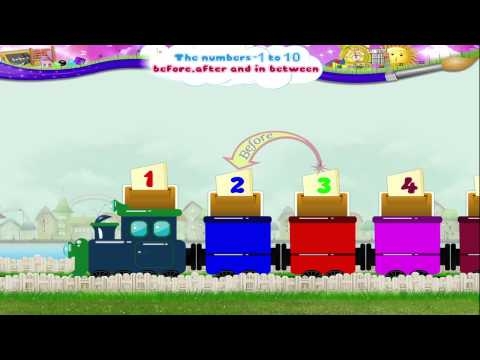## Hỗ trợ online

Hotline:
02862707677

Bài 6: Before, After and In between

(Trước, sau và ở giữa)

Các em làm quen với một số thuật ngữ cơ bản trong bài học "Before, after and In between" của môn Toán bằng Tiếng anh nhé

Bạn phải là "Học viên Trung Tâm Anh Ngữ Thần Đồng Việt" mới xem được Video!Bài 6: Before, After and In between

Starry, have you seen this train before?

It is called the number train.

Look at the number on the engine. It is the number 1.

The number that comes after the number 1 is the number 2.

After two, we have the number three.

And then four. And after 4 comes the number 5.

Follow closely by the number six. Next we have number 7.

After number 7, comes number 8.

And then number 9 join on the number train.

And finally, what is the number on the last compartment Starry? Yes, it is the number 10.

Isn’t it the colorful number train, Starry?

Now, that you have learned to find the number that comes after.

Let us try to find the number that comes before its number.

Now, the number that comes before ten is the number 9.

And the number that comes before 9 is number 8.

Starry, what is the number that comes before 8. Right, it is 7.

And of course the number that comes before 7 is 6.

Now five is the number that comes before six

and the number that comes before five is four.

Three comes before four. And two comes before three.

And finally the number that comes before two is the number one.

Wow, with that Starry.

You have learned to find out a number that comes before another number.

But Starry, what if I ask you what is the number that comes in between two numbers?

You look puzzled. It is easy.

Wait, let me explain.

Look at the number train, the number that comes between 1 and 3 is two.

The number that comes between four and six is five.

Isn’t that easy Starry?

Now, what would the number that comes between 7 and 9 be 8.

Right and of course.

The number that comes between 8 and 10 is 9.

Starry, shall we do an activity together.

Fill in the blanks with the correct numbers.

The number that comes before 8 is (dash).

The number that comes before 8 is 7.

The number that comes after 4 is (dash).

The number that comes after 4 is 5.

The number that comes before 3 is (dash).

The number that comes before 3 is 2.

The number that comes before 6 is (dash).

The number that comes before 6 is 5.

The number that comes after 8 is (dash).

The number that comes after 8 is 9.

The number that comes before 10 is (dash).

The number that comes before 10 is 9.

And in this activity, Starry, you have to fill in the missing number into the boxes.

Shall we begin. 2 (dash) 4.

The missing number is 3. 5 (dash) 7.

The missing number is 6. 8 (dash) 10.

The missing number is 9. 3 (dash) 5.

What do you think is the missing number Starry?

Yes, it is 4. 1 (dash) 3.

1,2,3. Correct!

The missing number is 2. 7 (dash) 9.

The missing number is 8. That was excellent, Starry!

Starry, with this number train, we have not only learned how to find out the number that come before and after particular number but also a number that is in between 2 numbers.